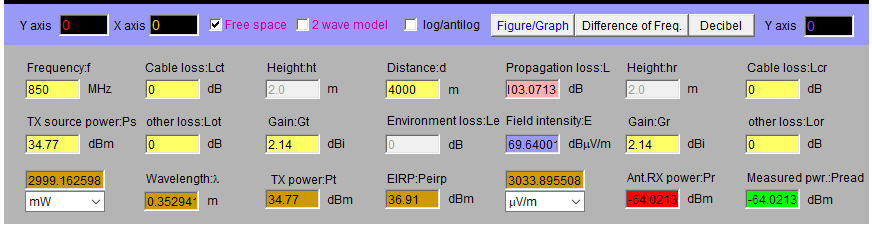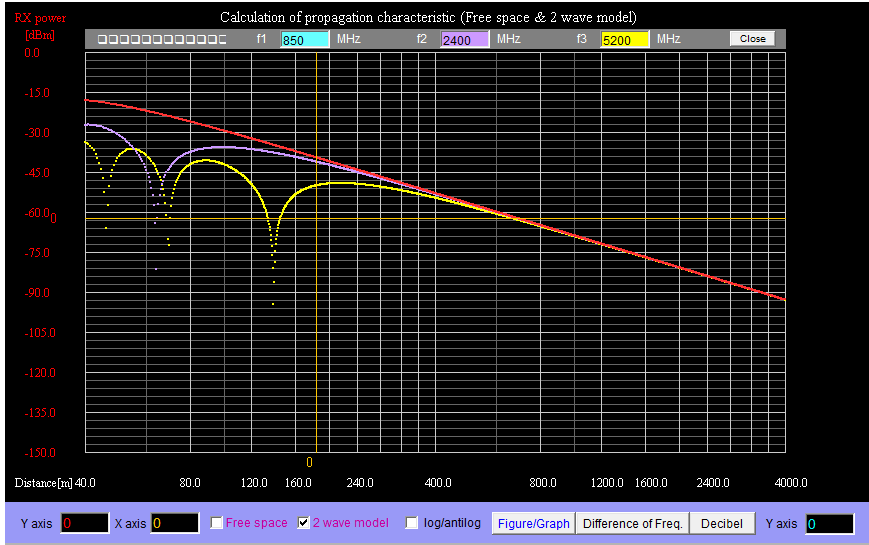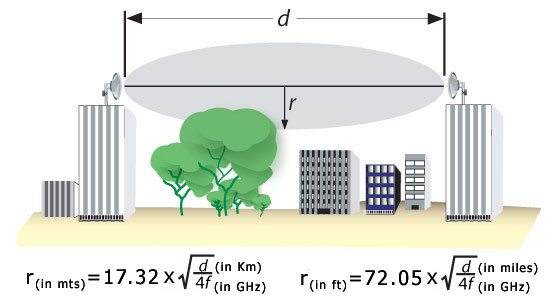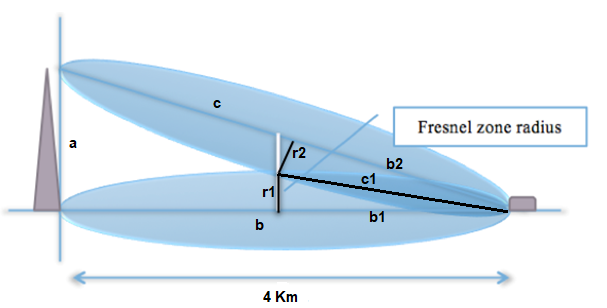## Lab 9 – Wireless Link Budget Estimation (II)

We will spend totally three weeks on the wireless link budget estimation task. You will continue to finish task 2 listed in this document if you haven’t got it done last week. More hints are provided in this document, please read through carefully.

You are planning for a WWAN deployment in a new suburb. For each residential area with the radius of 4km approximately, you plan to have one transmission station that provides LTE service using 850MHz band. On the receiver side, each house in the area is planned to be equipped with a Cisco LTE enable router that has EHWIC-4G-LTE-G card and Cisco 4G-LTE-ANTM-D dipole antenna installedTask 1: (for Week 8)

Your task is to perform link budget estimation to find out the minimum transmission power required by the transmission station so the whole area can be covered with the maximum transmission speed.  Assume that the transmission antenna is positioned high enough so the distance between the transmitter and receiver antennas is Light-of-Sight and there is no obstacle between them. The SNR to get maximum speed is 20dB and the transmitter antenna gain is 2dBi.

Solution.

According to given information in the task as they are

No Fresnel Zone effect

Distance between Transmitter and Receiver – 4Km

Frequency Band – 850Mhz

Transmitter antenna gain – 2dBi

SNR – 20dB

So according to the research and given value we have find out all the possible value for the table and they are as follow.

 Parameters Value Distances between Transmitter and Receiver 4Km Light-of-Sight Yes Fresnel zone effect No Frequency band 850Mhz Transmitter Antenna Gain 2dBi Receiver RSSI 25dB Receiver Antenna Gain 2dbi Required SNR 20db

First, we calculate the Received Power.  To do this, the antenna gains of the radio system and the transmitter power are needed to be gathered from the documented data sheet of the Cisco official site.

Since the transmission will be using the same device for transmission as well as receiver also. So the link budget of both the radios will be symmetric. After consulting the sheet given below are the obtained values.

Tx power =34.77 dBm

Internal antenna Gain = 2dBi

The Fresnel Zone effect is given as none in the table, so only FSPL is needed to be considered for the calculation of the received power. The FSPL at 4 km is 103.58dB for 900MHz as per the Table in the given document name Link_budget_Estimation. We may thereafter calculate the received power as follows:

Received Power (dBm) = Tx Antenna Gain (dBi) + Tx Power (dBm) + Rx Antenna Gain (dBi) – FSPL (dB)                                               = 2   +  34.77  +   2   - 103.58

= -64.81

To achieve the data rate of 54MBps, the SNR required is 25dB. The maximum channel noise can now be calculated as:

Maximum Channel Noise (dBm) = Received Power (dBm) – SNR (dB)

= -64.81 – 25

= -89.81

Now we need to calculate the Link margin:

= -64.81  -   (-72)

= 7.19

 Parameters Value Required transmission power -72 Maximum channel noise -89.81 Link margin 7.19

Go to the following URL

Input the required transmission power you have just calculated and other parameters to the link budget calculator. Leave all obstacles option as none.

1.What distance value do you get?

Solution. The distance value is 1.124Km

2.Is it bigger or smaller than 4km?

Solution.The value is smaller than the 4Km

3.Why do you think?

Solution.The value is small because of the less power utilized in the given antenna to transmit the signal from the source to destination.

Now try different obstacle types for obstacle1 option, and answer the following questions:

1.Which obstacle will reduce the distance value most?

Solution.The concrete wall and the metal door (Office Brick Wall) will have reduced the distance more almost around 0.282 Km.

2.Which obstacle will have less impact on the distance?

Solution.The Thin brick wall have the least effect on the signal strength almost around 0.893Km.

Now go to the following link: http://www.cdt21.com/resources/siryo5.asp

Input the frequency, distance, transmission power, antenna gains and FSPL values to the online calculator.

1.What received power do you get?

2.Is it what you are expected?

We get the -64.02 dBm for the received power as shown below.This is value is not we are expecting in the calculation of the signal strength.

2-wave propagation model for 850MHz, 2.4GHz and 5GHz frequencies.Can you explain why the propagation characteristics have the crests i.e. consecutive areas where signals are strong and become weak and then strong again etc.?

Solution.The characteristics shown in the graph is due to the signal wavelength. The higher frequency signal are inefficient in the smaller distances that’s why we see the signal strength drop. And there is no issue in the as the signal goes for the longer distances which is mostly likely the case used in the satellite communication.

### Lab 10 – Wireless Link Budget Estimation (III)

We will spend totally three weeks on the wireless link budget estimation task. You will continue to finish task 2 listed in this document if you haven’t got it done last week. More hints are provided in this document, please read through carefully.

You are planning for a WWAN deployment in a new suburb. For each residential area with the radius of 4km approximately, you plan to have one transmission station that provides LTE service using 850MHz band. On the receiver side, each house in the area is planned to be equipped with a Cisco LTE enable router that has EHWIC-4G-LTE-G card and Cisco 4G-LTE-ANTM-D dipole antenna installed

Your task is to perform link budget estimation to find out the minimum transmission power required by the transmission station so the whole area can be covered with the maximum transmission speed.  Assume that the transmission antenna is positioned high enough so the distance between the transmitter and receiver antennas is Light-of-Sight and there is no obstacle between them. The SNR to get maximum speed is 20dB and the transmitter antenna gain is 2dBi.

Solution.

According to given information in the task as they are

No Fresnel Zone effect

Distance between Transmitter and Receiver – 4Km

Frequency Band – 850Mhz

Transmitter antenna gain – 2dBi

SNR – 20dB

So according to the research and given value we have find out all the possible value for the table and they are as follow.

 Parameters Value Distances between Transmitter and Receiver 4Km Light-of-Sight Yes Fresnel zone effect No Frequency band 850Mhz Transmitter Antenna Gain 2dBi Receiver RSSI 25dB Receiver Antenna Gain 2dbi Required SNR 20db

Since the transmission will be using the same device for transmission as well as receiver also. So the link budget of both the radios will be symmetric. After consulting the sheet given below are the obtained values.

Tx power =34.77 dBm

Internal antenna Gain = 2dBi

The FSPL at 4 km is 103.58dB for 900MHz as per Table 1 in the given document name Link_budget_Estimation. The received power can then be calculated as follow:

Received Power (dBm) = Tx Power (dBm) + Tx Antenna Gain (dBi) + Rx Antenna Gain (dBi) – FSPL (dB)                                               = 34.77   +   2   +   2  - 103.58

= -64.81

Required SNR to achieve 54MBps data rate is 25dB, as per Table 3. Now, the maximum channel noise is:

Maximum Channel Noise (dBm) = Received Power (dBm) – SNR (dB)

= -64.81 – 25

= -89.81

Now we need to calculate the Link margin

=   -64.81   -  (-72)

=    7.19

 Parameters Value Required transmission power -72 Maximum channel noise -89.81 Link margin 7.19

Go to the following URL

Input the required transmission power you have just calculated and other parameters to the link budget calculator. Leave all obstacles option as none.

1.What distance value do you get?

Solution . The distance value is 1.124Km

2.Is it bigger or smaller than 4km?

Solution.The value is smaller than the 4Km

3.Why do you think?

Solution.The value is small because of the less power utilized in the given antenna to transmit the signal from the source to destination.

Now try different obstacle types for obstacle1 option, and answer the following questions:

3.Which obstacle will reduce the distance value most?

Solution.The concrete wall and the metal door (Office Brick Wall) will have reduced the distance more almost around 0.282 Km.

4.Which obstacle will have less impact on the distance?

Solution.The Thin brick wall have the least effect on the signal strength almost around 0.893Km.

Now go to the following link: http://www.cdt21.com/resources/siryo5.asp

Input the frequency, distance, transmission power, antenna gains and FSPL values to the online calculator.

3.What received power do you get?

4.Is it what you are expected?

We get the -64.02 dBm for the received power as shown below.This is value is not we are expecting in the calculation of the signal strength.

2-wave propagation model for 850MHz, 2.4GHz and 5GHz frequencies.Can you explain why the propagation characteristics have the crests i.e. consecutive areas where signals are strong and become weak and then strong again etc.?

Solution.The characteristics shown in the graph is due to the signal wavelength. The higher frequency signal are inefficient in the smaller distances that’s why we see the signal strength drop. And there is no issue in the as the signal goes for the longer distances. Which is mostly likely the case used in the satellite communication.

In the first lab, we have assumed that the transmission antenna is positioned high enough to avoid the Fresnel zone effect. In this task we will drop this assumption.

Your task now is to compute the minimum height required by the transmission antenna to safely avoid the effect of Fresnel zone. You can assume that there is no obstacle between the transmitter and the receiver antenna so the Fresnel zone effect is only due to the interference with the earth surface.

Solution.The required calculation to get required height of the transmission antenna is given below.

1.We need to compute the radius of the first Fresnel zone given the frequency and distance between the transmitter and the receiver. Using the given below Fresnel zone formula.r=17.32 × √(d÷4f)

=17.32 × √(4 ÷4 ×0.850)

= 15.968 meters

Now in order to determine the height of the tower we need to follow the following diagram.Now calculating the c1,

B1 is half of the b, so b1 = 2km

C1^2 = b1^2 + r1^2

= 4,000,000 + 254.977024

= 4,000,254.977024

= 2000.06374

cos? = b1/c1

= 2000/2000.06374

= 0.9999681

Or  in degree

= 0.4576507

Now since the value of the angle is double of the calculated value. Then the result will be

= 0.9153014

So, now

cos? = b/c

cos 0.9153014= 4000/c

0.9998724 = 4000/c

C = 4000/0.9998724

C = 4,000.5104651

Now c^2 = a^2 + b^2

4,000.5104651^2 = a^2 + 4000^2

A^2 = 4,000.5104651^2 – 4000^2

A^2 = 4083.90936543002712

A = 63.9054

So the height of the tower will be around 63.9054 meters.